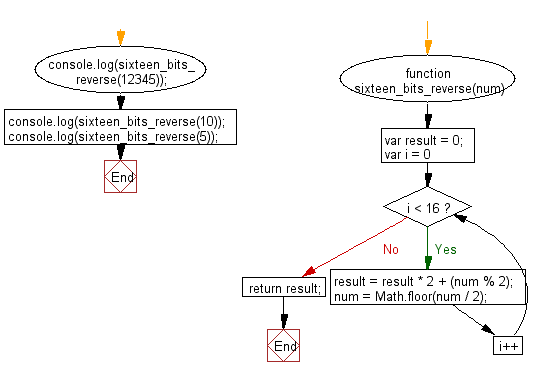# JavaScript: Reverse the bits of a given 16 bits unsigned short integer

## JavaScript Basic: Exercise-138 with Solution

Write a JavaScript program to reverse the bits of a given 16 bits unsigned short integer.

Sample Solution:

HTML Code:

``````<!DOCTYPE html>
<html>
<meta charset="utf-8">
<meta name="viewport" content="width=device-width">
<title>Reverse the bits of a given 16 bits unsigned short integer</title>
<body>

</body>
</html>
```
```

JavaScript Code:

``````function sixteen_bits_reverse(num) {
var result = 0;
for (var i = 0; i < 16; i++)
{
result = result * 2 + (num % 2);
num = Math.floor(num / 2);
}
return result;
}
console.log(sixteen_bits_reverse(12345));
console.log(sixteen_bits_reverse(10));
console.log(sixteen_bits_reverse(5));
``````

Sample Output:

```39948
20480
40960
```

Flowchart:ES6 Version:

``````function sixteen_bits_reverse(num) {
let result = 0;
for (let i = 0; i < 16; i++)
{
result = result * 2 + (num % 2);
num = Math.floor(num / 2);
}
return result;
}
console.log(sixteen_bits_reverse(12345));
console.log(sixteen_bits_reverse(10));
console.log(sixteen_bits_reverse(5));
``````

Live Demo:

See the Pen javascript-basic-exercise-137 by w3resource (@w3resource) on CodePen.

Improve this sample solution and post your code through Disqus

What is the difficulty level of this exercise?

Test your Programming skills with w3resource's quiz.

﻿

## JavaScript: Tips of the Day

Returns the sum of the powers of all the numbers from start to end (both inclusive)

Example:

```const sumPower = (end, power = 2, start = 1) =>
Array(end + 1 - start)
.fill(0)
.map((x, i) => (i + start) ** power)
.reduce((a, b) => a + b, 0);
console.log(sumPower(10)); // 385
console.log(sumPower(10, 3)); // 3025
console.log(sumPower(10, 3, 5)); // 2925
```

Output:

```385
3025
2925
```